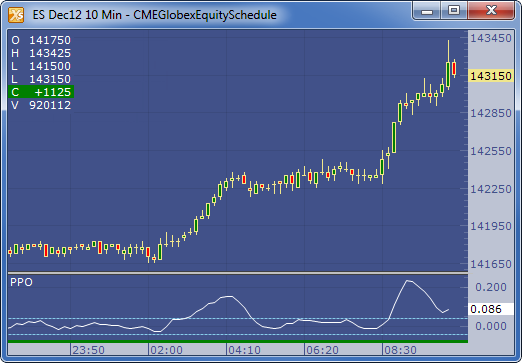← Back to X_TRADER® Help Library

### X_STUDY® Documentation

#### Percent Price Oscillator (PPO)

##### Description

The Percent Price Oscillator (PPO) is based on the differences between two moving averages of different lengths, a ‘Fast’ and a ‘Slow’ moving average. The PPO is the difference of the two averages divided by the slower of the two moving averages, which tends to normalize the values across different instruments.

##### Formula

PPO = ( ( FastMA - SlowMA ) / SlowMA ) * 100

Where:

•  FastMA is the shorter moving average and SlowMA is the longer moving average.
• When the FastMA is greater than the SlowMA, the PPO is a positive number, and when the FastMA is lower than the SlowMA, the PPO is a negative value.
##### Example